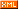# linux

 博客园 :: 首页 :: 博问 :: 闪存 :: 新随笔 :: 联系 :: 订阅:: 管理 ::__setup("para_name=", parse_func)

para_name 为参数名，parse_func 为分析参数值的函数，它负责把该参数的值转换成相应的内核变量的值并设置那个内核变量。内核为整数参数值的分析提供了函数 get_option 和 get_options，前者用于分析参数值为一个整数的情况，而后者用于分析参数值为逗号分割的一系列整数的情况，对于参数值为字符串的情况，需要开发者自定义相应的分析函数。在源代码包中的内核程序kern-boot-params.c 说明了三种情况的使用。该程序列举了参数为一个整数、逗号分割的整数串以及字符串三种情况，读者要想测试该程序，需要把该程序拷贝到要使用的内核的源码目录树的一个目录下，为了避免与内核其他部分混淆，作者建议在内核源码树的根目录下创建一个新目录，如 examples，然后把该程序拷贝到 examples 目录下并重新命名为 setup_example.c，并且为该目录创建一个 Makefile 文件：

obj-y = setup_example.o

Makefile 仅许这一行就足够了，然后需要修改源码树的根目录下的 Makefile文件的一行，把下面行

core-y          := usr/

core-y          := usr/ examples/

setup_example_int=1234 setup_example_int_array=100,200,300,400 setup_example_string=Thisisatest

setup_example_int=1234

setup_example_int_array=100,200,300,400

setup_example_int_array includes 4 intergers

setup_example_string=Thisisatest

//filename: kern-boot-params.c#include <linux/kernel.h>#include <linux/init.h>#include <linux/string.h>#define MAX_SIZE 5static int setup_example_int;static int setup_example_int_array[MAX_SIZE];static char setup_example_string;static int __init parse_int(char * s){        int ret;        ret = get_option(&s, &setup_example_int);        if (ret == 1) {                printk("setup_example_int=%d\n", setup_example_int);        }        return 1;}static int __init parse_int_string(char *s){        char * ret_str;        int i;        ret_str = get_options(s, MAX_SIZE, setup_example_int_array);        if (*ret_str != '\0') {                printk("incorrect setup_example_int_array paramters: %s\n", ret_str);        }        else {                printk("setup_example_int_array=");                for (i=1; i<MAX_SIZE; i++) {                        printk("%d", setup_example_int_array[i]);                        if (i < (MAX_SIZE -1)) {                                printk(",");                        }                }                printk("\n");                printk("setup_example_int_array includes %d intergers\n", setup_example_int_array);        }        return 1;}static int __init parse_string(char *s){        if (strlen(s) > 15) {                printk("Too long setup_example_string parameter, \n");                printk("maximum length is less than or equal to 15\n");        }        else {                memcpy(setup_example_string, s, strlen(s) + 1);                printk("setup_example_string=%s\n", setup_example_string);        }        return 1;}/*宏__setup()将分析参数的函数注册到内核中*/__setup("setup_example_int=", parse_int);__setup("setup_example_int_array=", parse_int_string);__setup("setup_example_string=", parse_string);
posted on 2011-04-10 15:33  h13  阅读(1613)  评论(0编辑  收藏  举报